Email us to get an instant 20% discount on highly effective K-12 Math & English kwizNET Programs!

#### Online Quiz (WorksheetABCD)

Questions Per Quiz = 2 4 6 8 10

### MEAP Preparation - Grade 4 Mathematics1.41 Types of Fractions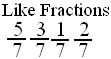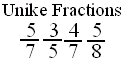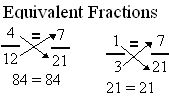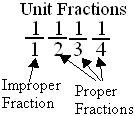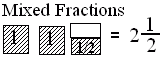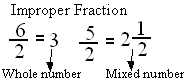Directions: Answer the following questions. Also write at least 5 examples of each type of fractions.
 Q 1: A mixed number denotes the sum of a whole number-part and proper fraction part.FalseTrue Q 2: Fraction with numerator 1 is called a unit fraction. Are all unit fractions proper?YesNo Q 3: If the numerator is more than the denominator in a fraction, the fraction is called a _________.improper fractionproper fractionmixed fraction Q 4: A number with a whole number and a proper fraction is amixed numberproper number Question 5: This question is available to subscribers only! Question 6: This question is available to subscribers only!